# [Scala MOOC I] Lec4: Types and Pattern Matching

## 4.1 - Objects Everywhere

scala is pure OO: every value is an obj, every operation is a method of obj.

`scala.Int` `scala.Boolean` maps to JVM standard primitive types.

Implement `Boolean` withous primitive type in scala: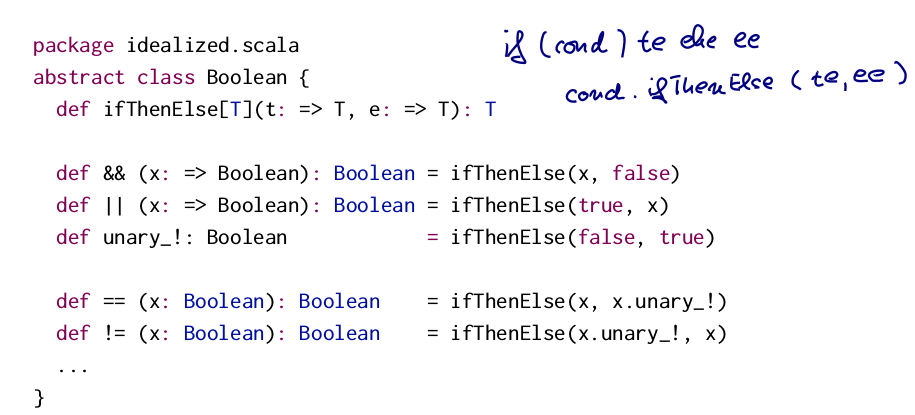Then defin `false` and `true` as objects, give implementation for `ifThenElse()` funciton:

```object true extends Boolean{
def ifThenElse[T](t: =>T, e: =>T) = t
}
object false extends Boolean{
def ifThenElse[T](t: =>T, e: =>T) = e
}
```

## 4.2 - Functions as Objects

Function values are treated as objects in scala.
the type `A => B` is an abbrevation for `scala.Function1[A, B]`Functions are objects with `apply` methods.

anonymous function `(x: Int) => x*x` is expanded to :

```new Function1[Int, Int]{
def apply(x: Int) = x*x
}
```

function call `f(a,b)` is expanded to: `f.apply(a,b)` `List(1,2)` is expanded to : `List.apply(1,2)`

## 4.3 - Subtyping and Generics

2 forms of polymorphism: subtyping and generics

### Type Bounds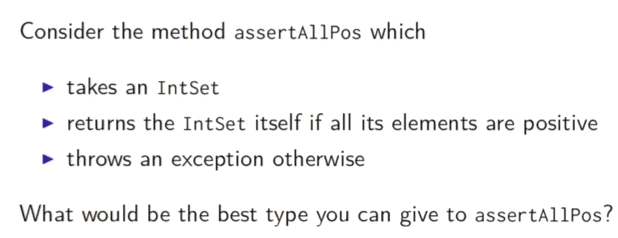`assertAllPos` taks either `EmptySet` or `NonEmptySet`, the return type is EmptySet (when para=EmptySet) or NonEmptySet (when para=NonEmpty).
To express this situation:

`def assertAllPos[S <: IntSet](r: S): S = ...`

Here `<: IntSet` means IntSet is an upper bound of the type parameter.and we can mix the two notations:

`[S >: NonEmpty <: IntSet]`

### Covariance

Given `NonEmpty <: IntSet`,
should we have: `List[NonEmpty] <: List[IntSet]` ?

In this case the typse are called *covariant. *

In java, arrays are covariant: `NonEmpty[] <: IntSet[]` but this might cause problems: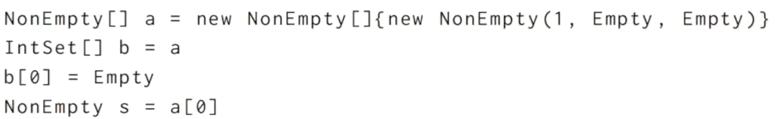will get ArrayStoreException in 3rd line.

Liskov substitution principle: when a type can be a subtype of another

If A<:B, then everything one can do with an object of type B, one should also be able to do it with object of type A.

In scala the Array is not covariant: `Array[IntSet]` is not supertype of `Array[NonEmpty]`

## 4.5 - Decomposition

ex: arithmetic expression interpreter: a tree of numbers and sums, both are subtype of `Expr`.

1st try: test and accessor methods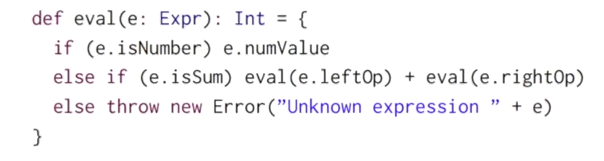→ tedious... And to add more operands need to add many more methods...

2nd try: (non-solution) type testing and type casting `isinstantceof`, `asinstanceof`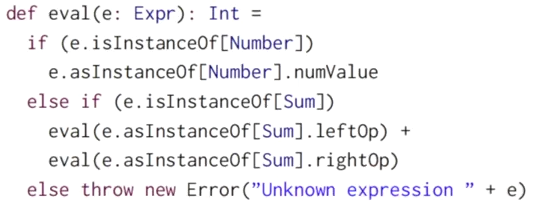discouraged in scala.

1st solution: OO decomposition add `eval` method to class Expr.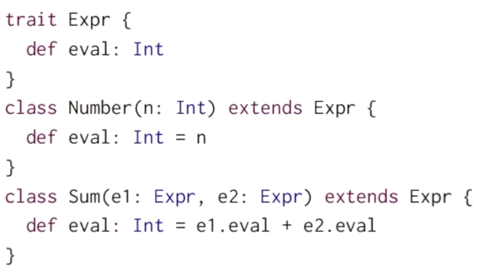limitation: if we want to simplify an expression,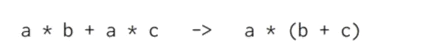⇒ there is no local simplification !

pattern matching

## 4.6 - Pattern Matching

goal: reverse the construction process.

• which subclass it is?
• what were the arguments?

### case classes

add `case` in class definition. ex.

```case class Number(n: Int) extends Expr
case class Sum(e1: Expr, e2: Expr) extends Expr
```

adding `case` adds companion objects with `apply` methods: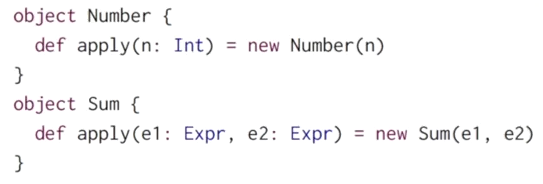according to the expansion, `Number(2)` is equal to `Number.apply(2)` which is actaully `new Numver(2)`

### pattern matching

`match`: extension of `switch` in java, ex:

```def eval(e: Expr): Int = e match{
case Number(n) => n
case Sum(e1, e2) => e1 + e2
}
```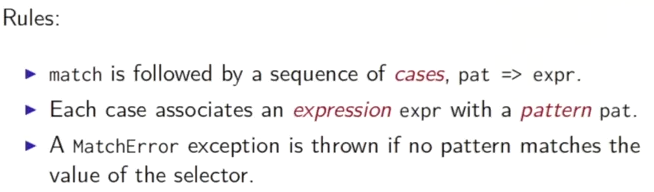patterns include:

• constructor, ex. `Number(n)`
• variable, ex. `n, e1, e2`
• wildcard patters, ex. `_`
• constant, ex. `1, true`
• or their combination, ex `Sum(Number(1), Var(x))`

The whole match expression is replaced with the expression to the rhs of the first match pattern.

exercice: implement a `show` function.

```def show(e: Expr): String = e match {
case Number(n) => n.toString
case Sum(l, r) => show(l) + "+" + show(r)
}
```

## 4.7 - Lists

example: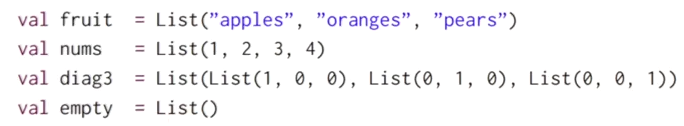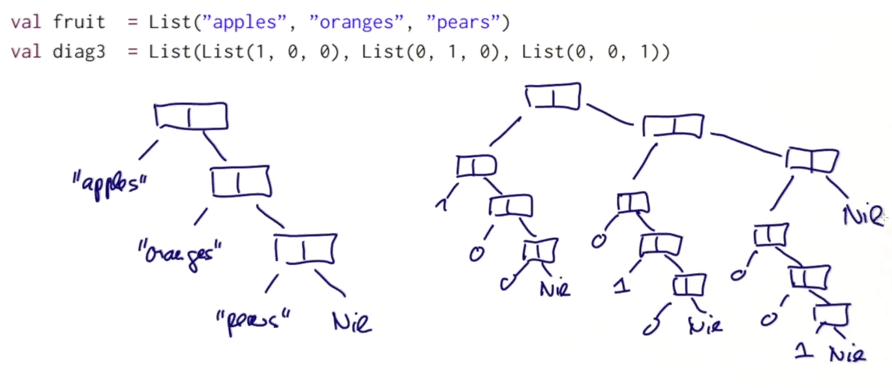difference between List and Array:

• Lists are immutable, elements cannot change
• Lists are recursive, arrays are flat
• both are homogeneous, all elements of the same type

All `List`s in scala are constructed with:

• Empty list `Nil`
• construction op `::`(pronunced: cons), `x::xs`convention in scala: operators ending with `:`

• are right-associative. `a::b::c` is equal to `a::(b::c)`
• seen as method calls from the right-hand operand. `1::2::Nil` equal to `Nil.::(2).::(1)` ( `::` is prepend operation)

operations on lists:

• tail
• isEmpty

list in pattern matching: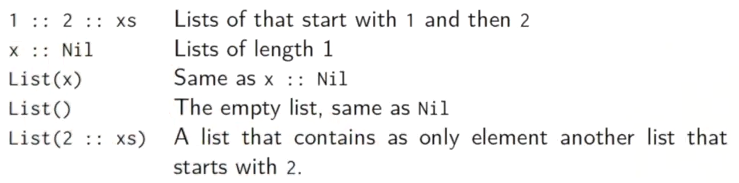ex. insertion sort of lists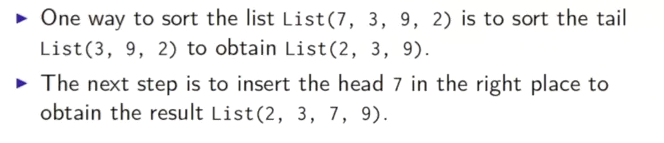```def isort(xs: List[Int]): List[Int] = xs match{
case List() => List()
case y::ys => insert(y, isort(ys))
}
def insert(x:Int, xs:List[Int]): List[Int] = xs match{
case List() => List(x)
case y::ys => if(x<=y) x::xs else y::insert(y, ys)
}
```